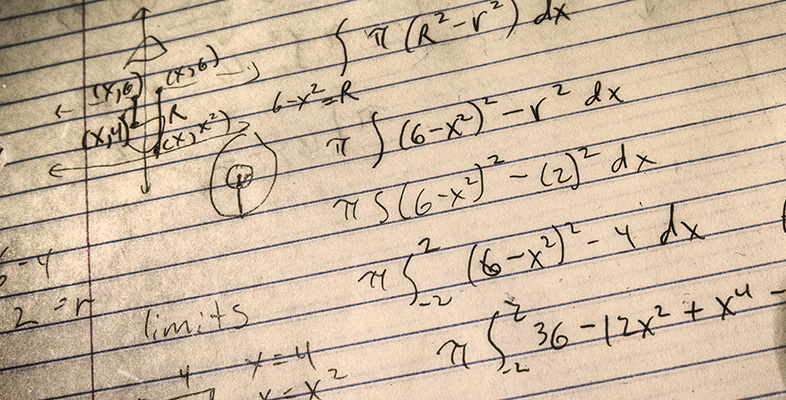An introduction to complex numbers

Start this free course now. Just create an account and sign in. Enrol and complete the course for a free statement of participation or digital badge if available.

Free course

# An introduction to complex numbers

## Introduction

This course is devoted solely to complex numbers.

In Section 1, we define complex numbers and show you how to manipulate them, stressing the similarities with the manipulation of real numbers.

Section 2 is devoted to the geometric representation of complex numbers. You will find that this is very useful in understanding the arithmetic properties introduced in Section 1.

In Section 3 we discuss methods of finding nth roots of complex numbers and the solutions of simple polynomial equations.

The final two sections deal with inequalities between real-valued expressions involving complex numbers. First we use inequalities in Section 4 to describe various subsets of the complex plane. Then we show, in Section 5, how to prove such inequalities. In particular, we introduce the Triangle Inequality, which can be used to obtain an estimate for the size of a given complex expression.

This OpenLearn course is an adapted extract from the Open University course M337 Complex analysis [Tip: hold Ctrl and click a link to open it in a new tab. (Hide tip)]

M337_1